Dehydration of alcohols

(I)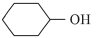(II)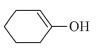(III)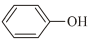(IV)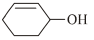will be in order:

1. I < II < III < IV

2. I > II > III > IV

3. III  < II < I < IV

4. II < III < IV < I

High Yielding Test Series + Question Bank - NEET 2020

Difficulty Level:

The reaction products of C6H5-O-CH3+ HI $\stackrel{\mathrm{A}}{\to }$

1. C6H5OH + CH3l

2. C6H5l + CH3OH

3. C6H5CH3 +HOI

4. C6H+ CH3Ol

High Yielding Test Series + Question Bank - NEET 2020

Difficulty Level: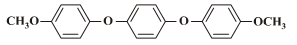How many moles of HI consumed in above reaction?

1. 1

2. 2

3. 3

4. 4

High Yielding Test Series + Question Bank - NEET 2020

Difficulty Level:

R-CH2-CH2OH can be converted into RCH2CH3COOH. The correct sequence of reagents is:

1. PBr3, KCN, H

2. PBr3, KCN, H2

3. KCN, H+

4. HCN, PBr3, H+

Concept Questions :-

Mechanism of Dehydration, Uses, Methanol and Ethanol
High Yielding Test Series + Question Bank - NEET 2020

Difficulty Level:

The Strongest acid among the following aromatic compunds is

1. p-chlorophenol

2. p-nitrophenol

3. m-nitrophenol

4. o- nitrophenol

Concept Questions :-

Phenols: Preparation, Properties, Acidic Nature of Phenol
High Yielding Test Series + Question Bank - NEET 2020

Difficulty Level:

(CH3)3C-O-CH3 + HI $\to$Products, the product are

1. (CH3)3C-OH + CH3l

2. (CH3)3C-l + CH3OH

3. (CH3)3C-l + CH3l

4. (CH3)3C-OH + CH3OH

Concept Questions :-

Ethers: Preparation, Physical & Chemical Properties, Uses
High Yielding Test Series + Question Bank - NEET 2020

Difficulty Level:

The reaction of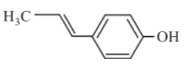with HBr gives

1.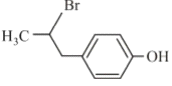2.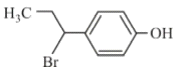3.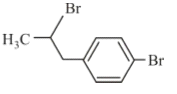4.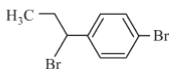Concept Questions :-

Electrophillic Substitution Reactions, Uses of Phenols
High Yielding Test Series + Question Bank - NEET 2020

Difficulty Level: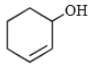$\underset{\mathrm{Acetone}}{\overset{{\left[{\left({\mathrm{CH}}_{3}\right)}_{3}\mathrm{CO}\right]}_{3}\mathrm{Al}}{\to }}X$

The product (x) of this reaction is

1.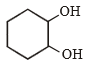2.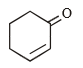3.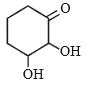4.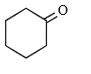Concept Questions :-

Alcohols: Preparation, Physical and Chemical Properties
High Yielding Test Series + Question Bank - NEET 2020

Difficulty Level:

CH3MgBr + Cyclopentanone $\stackrel{{H}_{2}O}{\to }$$\stackrel{HBr}{\to }$B$\stackrel{Mg/ether}{\to }$C$\underset{{H}_{3}O}{\overset{HCHO}{\to }}$D, D is

1.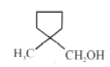2.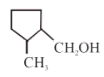3.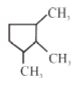4.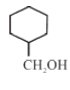High Yielding Test Series + Question Bank - NEET 2020

Difficulty Level: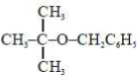can be repared from williamson's synthesis using

1.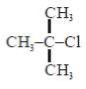and C26H5CH2ONa

2. C6H5CH2Cl and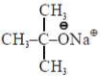3.and C6H5ONa

4. All of these